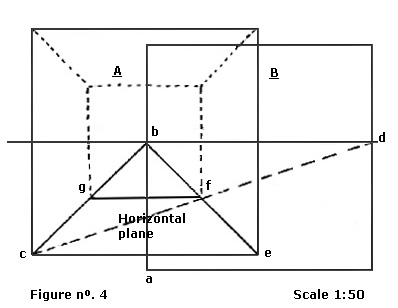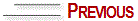# CONSTRUCTION OF THE SYSTEM

 1 — Construct square A drawn to scale. 2 — Calculate the distance of 17cm from the middle of square A base downwards. Determine point a. 3 — In the upward direction, draw a vertical line of 1.70m from bottom to top of point a and mark point b. 4 — Construct a square with the same dimensions of square A on the line constructed between points a and b. See square B in figure 4. 5 — Draw a horizontal line crossing the two squares at point b height. 6 — Draw a diagonal line from point c to point d (see figure 4). 7 — From the vertices of square A, draw lines that vanish in point b. 8 — Determine point f at the intersection of the lines that join points e, b, c and d. 9 — By joining points f and g with a horizontal line we will have a horizontal plane.

# NEW BASIC CONCEPT

The horizontal plane results from the overlapping of two images 17cm. apart from each other.© Yvonne Tessuto Tavares - All rights reserved